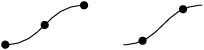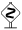# PyX — Example: path/at.py

0.4 KB
9.7 KB
0.8 KB
1.8 KB
1.1 KB

## Positions along a path```from pyx import *

def mark(x, y):
return path.circle(x, y, 0.1)

c = canvas.canvas()

p1 = path.curve(0, 0, 1, 0, 1, 1, 2, 1)
c.stroke(p1)
c.fill(mark(*p1.atbegin()))
c.fill(mark(*p1.at(0.5*p1.arclen())))
c.fill(mark(*p1.atend()))

p2 = path.curve(3, 0, 4, 0, 4, 1, 5, 1)
c.stroke(p2)
c.fill(mark(*p2.at(p2.begin()+0.5)))
c.fill(mark(*p2.at(p2.end()-0.5)))

c.writeEPSfile("at")
c.writePDFfile("at")
c.writeSVGfile("at")
```

### Description

There are several methods to access certain positions at a path. At first there are `atbegin` and `atend` methods, which return a coordinate tuple.In this example, we have defined a small helper function `mark` to which we can pass the return value of the `at...` methods. We do this by transforming the sequence in to positional argument of the function call. This Python language feature is available by the `*` syntax in the call.The coordinates returned by the `at...` methods are PyX lengths in the unscaleable true units similar to the return value of the `arclen` method.

For the left path `p1`, we also show how to use the `at` method, which can be used to get the coordinates of a certain point of the path depending on the arc length along the path.

A different use of the `at` function is shown for the right path `p2`: It is also possible to pass parametrization instances of the path to the `at` method. In the shown case we first fetch such parametrization instances for the beginning and the end of the path by the `begin` and `end` methods. Note that the `atbegin` method is equal to call `at` with the result value of the `begin` method (except for optimizations: atbegin is faster than the two calls). Similar `atend` could be rewritten using the result value of `end`. As shown in the example you can use the parametrization instances to add and substract arc lengths from selected point.The `at` method of a path instance can not only handle a single value, in which case it returns a single result tuple. You can also pass a list to the method and the return value will contain a list of coordinate tuples. You should bear in mind that passing a list is much more efficient than multiple calls of the method performing single conversions at each time.